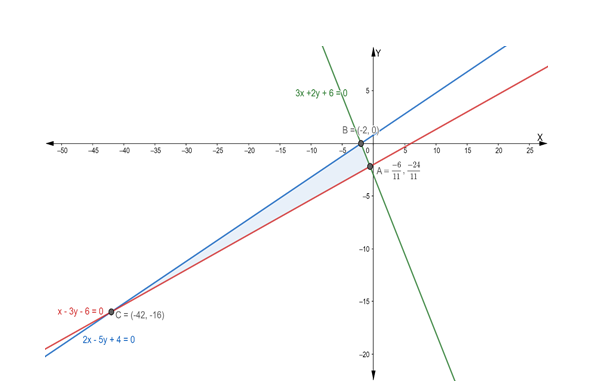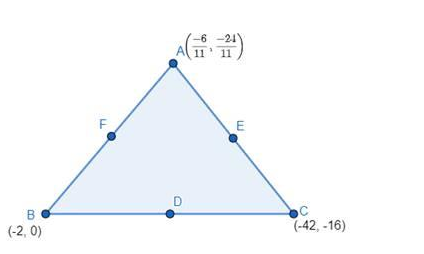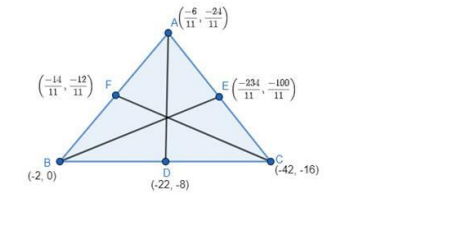# Find the equations of the medians of a triangle whose sides are given

Question:

Find the equations of the medians of a triangle whose sides are given by the equations 3x + 2y + 6 = 0, 2x – 5y + 4 = 0 and x -3y – 6 = 0.

Solution:

The given equations are

$3 x+2 y+6=0 \ldots$ (i)

$2 x-5 y+4=0 \ldots$ (ii)

and $x-3 y-6=0 \ldots$ (iii)

Let eq. (i), (ii) and (iii) represents the sides AB, BC and AC respectively of ΔABCFirstly, we solve the equation (i) and (ii)

$3 x+2 y+6=0 \ldots(i)$

$2 x-5 y+4=0 \ldots(i i)$

Multiplying the eq. (i) by 2 and (ii) by 3, we get

$6 x+4 y+12=0 \ldots A$

$6 x-15 y+12=0 \ldots B$

Subtracting eq. (B) from (A), we get

$6 x+4 y+12-6 x+15 y-12=0$

$\Rightarrow 19 y=0$

$\Rightarrow y=0$

Putting the value of y = 0 in eq. (i), we get

$3 x+2(0)+6=0$

$\Rightarrow 3 x+6=0$

$\Rightarrow 3 x=-6$

$\Rightarrow x=-2$

Thus, $A B$ and $B C$ intersect at $(-2,0)$

Now, we solve eq. (ii) and (iii)

$2 x-5 y+4=0 \ldots$ (ii)

and $x-3 y-6=0$.........(iii)

Multiplying the eq. (iii) by 2, we get

$2 x-6 y-12=0 \ldots$ (iv)

Subtracting eq. (iv) from (ii), we get

$2 x-5 y+4-2 x+6 y+12=0$

$\Rightarrow y+16=0$

$\Rightarrow y=-16$

Putting the value of y = -16 in eq. (ii), we get

$2 x-5(-16)+4=0$

$\Rightarrow 2 x+80+4=0$

$\Rightarrow 2 x+84=0$

$\Rightarrow 2 x=-84$

$\Rightarrow x=-42$

Thus, $B C$ and $A C$ intersect at $(-42,-16)$

Now, we solve eq. (iii) and (i)

$x-3 y-6=0 \ldots($ iii $)$

$3 x+2 y+6=0 \ldots($ i)

Multiplying the eq. (iii) by 3, we get

$3 x-9 y-18=0 \ldots(v)$

Subtracting eq. (v) from (i), we get

$3 x+2 y+6-3 x+9 y+18=0$

$\Rightarrow 11 y+24=0$

$\Rightarrow 11 y=-24$

$\Rightarrow \mathrm{y}=-\frac{24}{11}$

Putting the value of y in eq. (iii), we get

$x-3\left(-\frac{24}{11}\right)-6=0$

$\Rightarrow x+\frac{72}{11}-6=0$

$\Rightarrow x=6-\frac{72}{11}$

$\Rightarrow x=\frac{66-72}{11}$

$\Rightarrow x=-\frac{6}{11}$

Thus, $\mathrm{AC}$ and $\mathrm{AB}$ intersect at $\left(-\frac{6}{11},-\frac{24}{11}\right)$

So, vertices of triangle $\mathrm{ABC}$ are : $\mathrm{A}\left(-\frac{6}{11},-\frac{24}{11}\right), \mathrm{B}(-2,0) \& \mathrm{C}(-42,-16)$

Let D, E and F be the midpoints of sides BC, CA and AB respectively.Then the coordinates of D, E and F are

Coordinates of $D=\left(\frac{-42+(-2)}{2}, \frac{-16+0}{2}\right)$

$=\left(\frac{-42-2}{2},-\frac{16}{2}\right)$

$=\left(-\frac{44}{2},-8\right)$

$=(-22,-8)$

Coordinates of $E=\left(\frac{-42+\left(-\frac{6}{11}\right)}{2}, \frac{-16+\left(-\frac{24}{11}\right)}{2}\right)$

$=\left(\frac{-42-\frac{6}{11}}{2}, \frac{-16-\frac{24}{11}}{2}\right)$

$=\left(\frac{-462-6}{22}, \frac{-176-24}{22}\right)$

$=\left(-\frac{468}{22},-\frac{200}{22}\right)$

$=\left(-\frac{234}{11},-\frac{100}{11}\right)$

Coordinates of $F=\left(\frac{-\frac{6}{11}+(-2)}{2}, \frac{-\frac{24}{11}+0}{2}\right)$

$=\left(\frac{-6-22}{22},-\frac{24}{22}\right)$

$=\left(-\frac{28}{22},-\frac{12}{11}\right)$

$=\left(-\frac{14}{11},-\frac{12}{11}\right)$

Now, we have to find the equations of Medians AD, BE and CFThe equation of median AD is

$y-\left(-\frac{24}{11}\right)=\frac{-8-\left(-\frac{24}{11}\right)}{-22-\left(-\frac{6}{11}\right)}\left[x-\left(-\frac{6}{11}\right)\right]$

$\Rightarrow \mathrm{y}+\frac{24}{11}=\frac{\frac{-88+24}{11}}{\frac{-222+6}{11}\left(\mathrm{x}+\frac{6}{11}\right)}$

$\Rightarrow \mathrm{y}+\frac{24}{11}=\frac{-64}{-216}\left(\mathrm{x}+\frac{6}{11}\right)$

$\Rightarrow \mathrm{y}+\frac{24}{11}=\frac{16}{59}\left(\mathrm{x}+\frac{6}{11}\right)$

$\Rightarrow \mathrm{y}+\frac{24}{11}=\frac{16}{59} \mathrm{x}+\frac{96}{59 \times 11}$

$\Rightarrow \frac{16}{59} \mathrm{x}-\mathrm{y}=\frac{24}{11}-\frac{96}{59 \times 11}$

$\Rightarrow \frac{16 \mathrm{x}-59 \mathrm{y}}{59}=\frac{1416-96}{59 \times 11}$

$\Rightarrow 16 \mathrm{x}-59 \mathrm{y}=\frac{1320}{11}$

$\Rightarrow 16 x-59 y=120$

The equation of the median BE is

$y-(0)=\frac{-\frac{100}{11}-0}{-\frac{234}{11}-(-2)}[x-(-2)]$

$\Rightarrow \mathrm{y}=\frac{-\frac{100}{11}}{\frac{-234+2}{11}}(\mathrm{x}+2)$

$\Rightarrow \mathrm{y}=\frac{-100}{-232}(\mathrm{x}+2)$

$\Rightarrow \mathrm{y}=\frac{25}{58}(\mathrm{x}+2)$

$\Rightarrow 58 y=25 x+50$

$\Rightarrow 25 x-58 y+50=0$

The equation of median AD is

$y-(-16)=\frac{-16-\left(-\frac{12}{11}\right)}{-42-\left(-\frac{14}{11}\right)}[x-(-42)]$

$\Rightarrow \mathrm{y}+16=\frac{\frac{-176+12}{11}}{\frac{-462+14}{11}}(\mathrm{x}+42)$

$\Rightarrow \mathrm{y}+16=\frac{-164}{-448}(\mathrm{x}+42)$

$\Rightarrow \mathrm{y}+16=\frac{41}{112}(\mathrm{x}+42)$

$\Rightarrow 112 y+1792=41 x+1722$

$\Rightarrow 41 x-112 y+1722-1792=0$

$\Rightarrow 41 x-112 y-70=0$